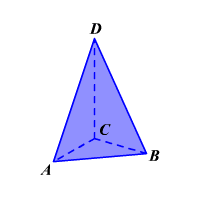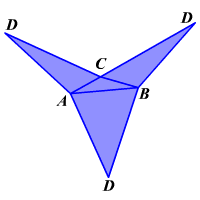# NETS

A two dimensional figure that, when folded, forms the surfaces of a three dimensional object.

Nets can be made for any solid figures. A net is a way of representing a polyhedron in two dimensions.

Look at the three dimensional figure shown.The figure $ABCD$ is a triangular pyramid . The base of the pyramid is $ABC$ .

The net of the triangular pyramid is shown. The net is a two dimensional figure that, when folded, forms the surfaces of the triangular pyramid.